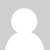### Arduino and RFM12B connections

Hi,

I have a question related to http://openenergymonitor.org/emon/sites/default/files/Cookbook_RFM12B_connections.png

I have the source DC of 3,6V to power arduino.

I'm going to drop voltage level to power the rfm12b to 3,3, but in this case what value should the 4,7kOm resistors have?

These values comes from 5v level , but If the arduino is powered with 3,6V, should I use the 4,7kOm or less?

Regards,

Jakub

### Re: Arduino and RFM12B connections

To get  a similar voltage level at the RFM12B, I would reduce the 4K7 resistors to 680 ohms or maybe 560 ohms.

```(10 / (10 + 0.68 )) * 3.6 = 3.37  // 680R with a 3.6V supply

(10 / (10 + 4.7 )) * 5 = 3.40     // 4K7 with a 5V supply

(10 / (10 + 0.56 )) * 3.6 = 3.41  // 560R with a 3.6V supply```### Re: Arduino and RFM12B connections

Thank you for calculations :)

### Re: Arduino and RFM12B connections

Hi!

If the 3,6V are stabilized then it is possible to use the RFM12B module without resistors.

According to the datasheet the supply voltage can be 2,2-3,8V => http://www.hoperf.com/upload/rf/RFm12b.pdf